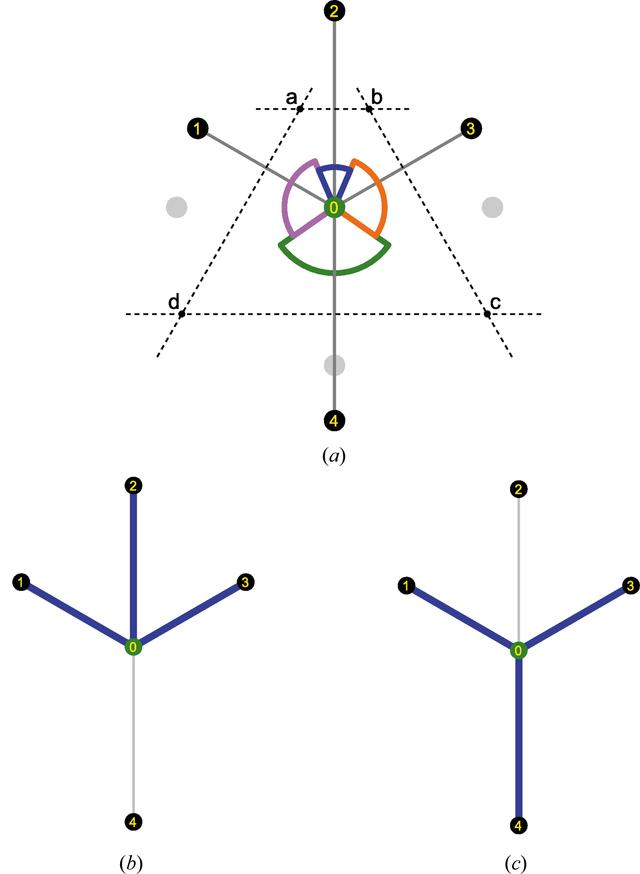disable zoom     view article Figure 10 Illustration in two dimensions of two sets of neighbors having the same coordination number. (a) Local environment of atom 0. Normalized distances to neighbors 1, 2, 3 and 4 are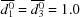,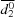= 1.15 and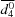= 1.35. Normalized angles to neighbors 1, 2, 3 and 4 are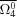= 1.0,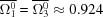and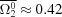. (b) Set of neighbors (1, 2 and 3) of atom 0 with N = 3. This set of neighbors is obtained with e.g. κ = 1.25 and γ = 0.3 cut-offs. (c) Another set of neighbors (1, 3 and 4) of atom 0 with N = 3. This set of neighbors is obtained with e.g. κ = 1.4 and γ = 0.5 cut-offs.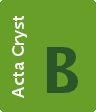STRUCTURAL SCIENCECRYSTAL ENGINEERINGMATERIALS
ISSN: 2052-5206
Volume 76| Part 4| August 2020| Pages 683-695